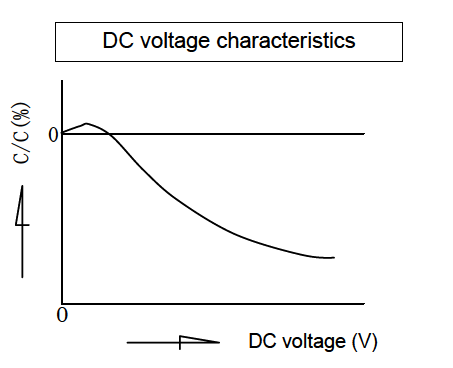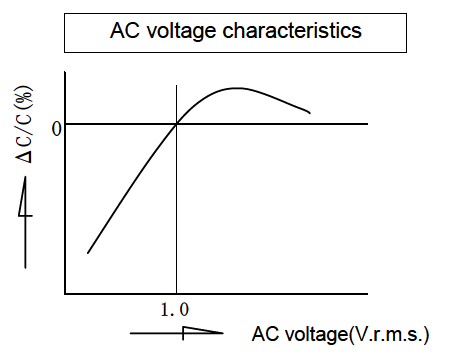# DC voltage and AC voltage characteristics

Safety Application Guide for Multilayer Ceramic Chip Capacitors
Design
Electrical factors
DC voltage and AC voltage characteristics

The capacitance of a capacitor changes depending on the DC voltage applied.Select a capacitor considering the DC voltage characteristics of the DC circuit in which the capacitor is used.

#### The capacitance of ceramic capacitors might change sharply depending on the applied voltage. (See figure)Confirm the followings in order to ensure desired capacitance.1. (1) Confirm whether the capacitance change according to the applied voltage is within an allowable range or not.
2. (2) Regarding DC voltage characteristics, capacitance decreases as voltage increases, even if the applied voltage is below the rated voltage.
Therefore, when a capacitor is used in a circuit with a narrow range of capacitance change
allowance like a time constant circuit, it is recommended that the capacitance is within the allowable range under operating voltage.

The capacitance of capacitor changes depending on the AC voltage applied. Select a capacitor considering the AC voltage characteristics of the AC circuit in which the capacitor is used.

#### The capacitance of ceramic capacitors might change depending on the applied voltage. (See figure) Confirm the followings in order to secure the capacitance1. (1) Confirm the capacitance change according to the applied voltage is within an allowable range.
2. (2) Confirm the measuring conditions of the capacitance specified in the catalogs or product specifications.
Capacitance during use may differ from the nominal capacitance.

Note: See Measurement of capacitance